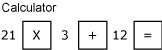### Become an OU studentFundamentals of accounting

Start this free course now. Just create an account and sign in. Enrol and complete the course for a free statement of participation or digital badge if available.

# 2.2 Use of BODMAS and brackets

When several operations are combined, the order in which they are performed is important. For example, 12 + 21 x 3 might be interpreted in two different ways:

(a) add 12 to 21 and then multiply the result by 3

(b) multiply 21 by 3 and then add the result to 12.

The first way gives a result of 99 and the second a result of 75. We need some way of ensuring that only one possible interpretation can be placed upon the formula presented. For this we use BODMAS, which give us the correct sequence of operations to follow so that we always get the right answer:

(B)rackets

(O)rder

(D)ivision

(M)ultiplication

(A)ddition

(S)ubtraction.

According to BODMAS, multiplication should always be done before addition, therefore 75 is actually the correct answer using BODMAS.

(‘Order’ may be an unfamiliar term to you in this context but it is merely an alternative for the more common term, ‘power’, which means a number is multiplied by itself one or more times. The ‘power’ of one means that a number is multiplied by itself once, i.e., 2 x 1, 3 x 1, etc., the ‘power’ of two means that a number is multiplied by itself twice, i.e., 2 x 2, 3 x 3, etc. In mathematics, however, instead of writing 3 x 3, we write 32 and express this as three to the ‘power’ or ‘order’ of 2.)

Brackets are the first term used in BODMAS and should always be used to avoid any possibility of ambiguity or misunderstanding. A better way of writing 12 + 21 x 3 is thus 12 + (21 x 3). This makes it clear which operation should be done first.

12 + (21 x 3) is thus done on the calculator by keying in 21 x 3 first in the sequence:Figure 1 Using BODMAS in a calculator

The next activity will test your ability to use brackets properly.

## Activity 1 Use of BODMAS

Complete the following calculations.

1. (13 x 3) + 17
To use this interactive functionality a free OU account is required. Sign in or register.
Interactive feature not available in single page view (see it in standard view).

56

1. (15 / 5) - 2
To use this interactive functionality a free OU account is required. Sign in or register.
Interactive feature not available in single page view (see it in standard view).

1

1. (12 x 3) / 2
To use this interactive functionality a free OU account is required. Sign in or register.
Interactive feature not available in single page view (see it in standard view).

18

1. 17 – (3 x (2 + 3))
To use this interactive functionality a free OU account is required. Sign in or register.
Interactive feature not available in single page view (see it in standard view).

2 (Did you enter the expression in the inner brackets i.e. (2 + 3) first?)

1. (13 + 2) / 3 - 4
To use this interactive functionality a free OU account is required. Sign in or register.
Interactive feature not available in single page view (see it in standard view).